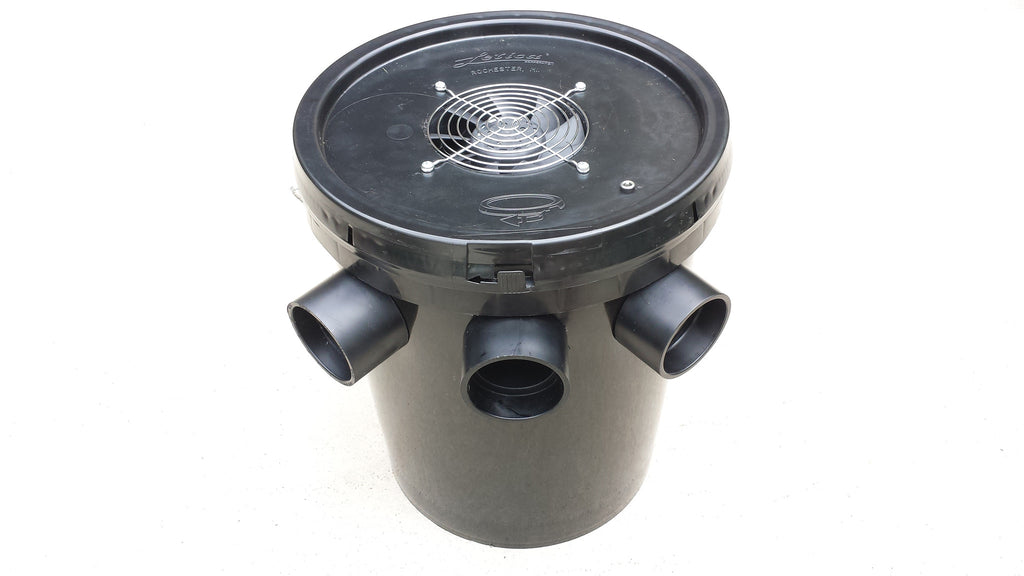# 5 Gallon Air Conditioner - Does it work? Battery Life

So here at 5gallonairconditioner.com, I keep getting asked about batteries, and how long they will last etc.

So, I will take a little break from the "does it work" series, to battery life, and battery life calculation to answer the "how long" question.

When talking about DC powered devices, there are 2 things to remember about "power," namely the quantity and how fast. So, how much power, and how fast is it coming, so to speak.

In electricity terms, these are called  AMPS and VOLTS.  Volts describes the how fast, and amps describe how much.

Think of electrical current this way. Think of a river, let's say a BIG river, like the Mississippi river. Let's say that the river flows at 10 miles per hour.  But, because the river is so BIG, it flows 600,000 cubic feet per second.

Now, lets compare that to a small fishing stream in Minnesota, that also flows at 10 miles per hour. But because of it's size, it only flows 500 cubic feet per second.

In this analogy, both rivers flow at 10 mph. But there is a huge difference in the QUANTITY of water that flows.

That is the same with electricity.  There are many common DC (Direct Current) batteries on the market.  There are AA, AAA, C, D, Car Batteries, Marine and deep cycle batteries, rechargeable batteries, etc.  So, for now, we will focus this discussion on 12V batteries.

In a 12V battery, the voltage rating is 12V, in our analogy, this is equivalent to 10mph.  So, the big question is current, or in electrical terms, the Amperage.

In the current model 5 gallon bucket air conditioners that are being sold, the current draw is approximately 4.5 amps.  What that means is that the fan takes 12V and 4.5 amps to work properly.  Remember, you need both, voltage AND amperage numbers to make an accurate "power" assessment.

So, the power consumed by these air conditioners is 12V and 4.5 amps.

So, electrical "power" is called WATTS.  Watts is simply the voltage multiplied by the amperage.  So, in these air conditioners, they take 54 WATTS to run them. Where 12*4.5=54.

So now that we know the power consumption, we can then calculate how much power we will need to run the device, and for how long the device will run by battery power.

So, for a typical alkaline D cell battery, it has both a voltage rating and an AMP-HOUR rating. So, now an amp-hour is simply how many amps over how many hours.  So, in our D cell example, it has a 1.5V and 17ah rating.

So, some quick calculations show, that if you have 8 D cell batteries hooked in series they will add up to 12V, and here's the tricky part, you don't add the AMP-HR ratings, they stay the same when the batteries are hooked in series. So, 8 D cell batteries hooked in series will make 12V and have 17ah of capacity.

So, now let's go back to the 5 gallon bucket air conditioner.  It takes 12V to run the fan, and the fan draws 4.5 amps of current (actually less, but for our example, that's what we'll use). So, if you hook up 8 D cell batteries, how long will the fan run?

Very simply, divide the 17ah rating of the batteries by the 4.5 amps of current draw of the fan, and that comes to approximately 3.8 hours.

So, there is the answer to the HOW LONG question.  If you hook 8 D cell alkaline batteries in series, in the 100% efficient case, the fan will run for 3.8 hours before the batteries will need to be changed.  In all practicality, with inefficiencies and battery age and battery temperature etc., 3 to 3.5 hours is probably more accurate.

So, when choosing a battery to run your new 5 gallon bucket air conditioner, a quick and dirty way to answer the "how long" question is to look at the AMP-HOUR rating of the 12V battery, and then divide the AH number by 5, and then that will give you the approximate number of hours that battery will run the fan before the batteries need to be replaced or recharged.

But, please remember, that the AH rating of a battery, is until "total discharge" so that means, as everyone from a common sense perspective knows, that even though the battery has an AH rating, that doesn't mean that the battery will have a "full charge" throughout that entire time frame.  Every battery has a different discharge profile, so even though the battery life may calculate out to 3 hours, that doesn't mean that the fan will be blowing "full speed" over that entire 3 hour period.

That "discharge profile" will be the subject of another blog post in the future, but for our purposes at this point, just remember that the device has a 4 amp power draw, and you can calculate the total battery life question, by simply dividing the battery AH rating by 5, to get a "ball park" estimate of battery life hours.